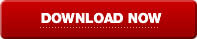# Decimal Odds Explained

Decimal Odds is the system used to display all prices on Betfair. Prices range from 1.01 to 1000. A Decimal Odds system also has many more increments between prices than a fractional odds system. To translate fractional odds to decimal odds, divide the fraction so that it becomes a decimal number and then add 1. For example 4/1 is 4 divided by 1 which is 4 then add 1 which equals 5.0. 9/4 is 9 divided by 4 which is 2.25 then add 1 so that your Decimal Odds for this is 3.25.

Our sports betting software can perform advanced odds calculations and be personalised to suit your preferences.

"A great piece of software, highly recommended."